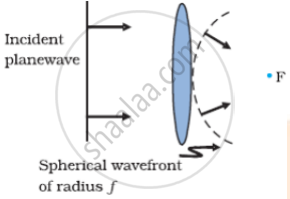# Define a Wavefront. Using 'Huygens' Principle, Draw the Shape of a Refracted Wavefront, When a Plane Wave is Incident on a Convex Lens. - Physics

Short Note

Define a wavefront. Using 'Huygens' principle, draw the shape of a refracted wavefront, when a plane wave is incident on a convex lens.

#### Solution

The locus of points, which oscillate in phase is called a wavefront; thus a wavefront is defined as a surface of constant phase.In the given figure we consider a plane wave incident on a thin convex lens, the emerging wavefront is spherical and converges to point F which is known as the focus.

Is there an error in this question or solution?
2014-2015 (March) Ajmer Set 2

Share